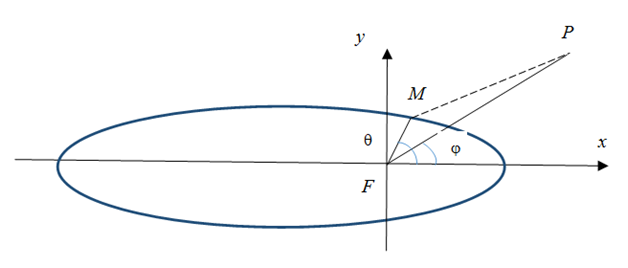###### eISSN: 2576-4543# Physics & Astronomy International JournalMini Review Volume 2 Issue 4

# Gravitional potential generated by an arc bent into an elliptical shape

#### Nour Eddine Najid function clickButton(){ var name=document.getElementById('name').value; var descr=document.getElementById('descr').value; var unCopyslNo=document.getElementById('unCopyslNo').value; document.getElementById("mydiv").style.display = "none"; $.ajax({ type:"post", url:"https://medcraveonline.com/captchaCode/server_action", data: { 'name' :name, 'descr' :descr, 'unCopyslNo': unCopyslNo }, cache:false, success: function (html) { //alert('Data Send');$('#msg').html(html); } }); return false; } Verify Captcha Regret for the inconvenience: we are taking measures to prevent fraudulent form submissions by extractors and page crawlers. Please type the correct Captcha word to see email ID.function refreshPage(){ $("#mydiv").load(location.href + " #mydiv"); }$(document).ready(function () { //Disable cut copy paste $('#msg').bind('cut copy paste', function (e) { e.preventDefault(); }); //Disable mouse right click$("#msg").on("contextmenu",function(e){ return false; }); }); .noselect { -webkit-touch-callout: none; /* iOS Safari */ -webkit-user-select: none; /* Safari */ -khtml-user-select: none; /* Konqueror HTML */ -moz-user-select: none; /* Firefox */ -ms-user-select: none; /* Internet Explorer/Edge */ user-select: none; /* Non-prefixed version, currently supported by Chrome and Opera */ cursor: none; }

Faculty of Sciences, University Hassan II Casablanca, Morocco

Correspondence: Nour Eddine Najid, Faculty of Sciences, University Hassan II Casablanca, BP 5366, Ma, Tel 0021?2522?2306?80, Fax 0021?2522?2306?74

Received: April 25, 2018 | Published: July 12, 2018

Citation: Najid NE. Gravitional potential generated by an arc bent into an elliptical shape. Phys Astron Int J. 2018;2(4):297-298. DOI: 10.15406/paij.2018.02.00102

# Abstract

In this Study we plane to calculate the gravitational potential generated by a ring bent into an elliptical mass distribution. In a first part we suppose that the mass distribution is homogeneous, after we take the case in which the mass distribution is inhomogeneous or anisotropic. The aim of this study is to generalise that of circular case and work out the behaviour of a test particle in the vicinity of that distribution. The rings around asteroids are recently discovered. This study will have an impact concerning the behaviour of a test particle moving around these distributions.

Keywords: potential–elliptic functions–gravitation, static case, dynamical case, anisotropic distribution, mass

# Introduction

In an earlier work Najid et al.,1 we studied the gravitational potential generated by an inhomogeneous asteroid with a parabolic mass distribution. We established the close form of this potential. It allows us to study both, the static and dynamical case in which a test particle is near the distribution.

In another work Najid et al.,2 we studied the case of a circular ring with an anisotropic distribution of mass. The mass distribution depends on the direction of observation. We manage to work out the problem in terms of elliptic functions.

In this work, we plane to generalise the case of ring by scrutinizing a more realistic case. In general, around all structures the rings are elliptical, and even with inhomogeneous or anisotropic mass distribution. In the literature we find studies like ellipsoids, material points and a segment of double material were used.3,4 Fred et al.,5 1982established the expressions for both the potential and the field of a disk. They suggested a formula of a ring. Anisotropic and inhomgeneous distributions are our new ideas.

# Gravitational potential generated by elliptical distribution in its plane

We will express the potential dV in a point P(x,y) generated by the infinitesimal point M of mass dm in the plane of the ellipse (Figure 1)Figure 1 Gravitational potential generated by elliptical distribution at plane.

dV is given by:

$dV=-G\frac{dm}{‖\stackrel{\to }{MP}‖}$

with

$FM=r$ and $FP=\rho$ .
$\left(r,\theta \right)$ are the polar coordinates of M.
$\left(\rho ,\phi \right)$ are the polar coordinates of P.
$\stackrel{\to }{MP}=\stackrel{\to }{FP}-\stackrel{\to }{FM}$ .
$dm=\lambda rd\theta$ , $\lambda$ is the linear mass of the ellipse.

We deduce

$dV=-G\frac{\lambda rd\theta }{\sqrt{{r}^{2}+{\rho }^{2}-2\rho rcos\left(\theta -\phi \right)}}$

# The origin of the coordinates is at the focus

If we take the origin at the focus F, we are getting :

$r=\frac{a\left(1-{e}^{2}\right)}{1+ecos\theta }$

Where a is the semi axis of the ellipse and e is the eccentricity

$V=-Ga\left(1-{e}^{2}\right)\underset{\theta =0}{\overset{\theta =2\pi }{\int }}\frac{\lambda \left(\theta \right)d\theta }{\sqrt{{a}^{2}{\left(1-{e}^{2}\right)}^{2}+{\rho }^{2}{\left(1+ecos\theta \right)}^{2}-2\rho a\left(1-{e}^{2}\right)\left(1+ecos\theta \right)\text{cos}\left(\text{θ}-\text{φ}\right)}}$

# The origin of the coordinates is at the center

In this case r is given by (Figure 2)

$r=\frac{b}{\sqrt{1-{e}^{2}co{s}^{2}\theta }}$Figure 2 Gravitational potential generated by elliptical distribution at origin.

b is the semi–minor axis of the ellipse.
In this case
$OM=r$ and $OP=\rho$
$\left(r,\theta \right)$ are the polar coordinates of M.
$\left(\rho ,\phi \right)$ are the polar coordinates of P
$\stackrel{\to }{MP}=\stackrel{\to }{OP}-\stackrel{\to }{OM}$

In this case we get :

$dV=-Gb\frac{\lambda \left(\theta \right)d\theta }{\sqrt{{b}^{2}+{\rho }^{2}\left(1-{e}^{2}co{s}^{2}\theta \right)-2b\rho \text{cos}\left(\theta -\phi \right)\sqrt{1-{e}^{2}co{s}^{2}\theta }}}$

Simplified solution–Particular case.2 In the case of a circular ring of radius a and $\lambda \left(\theta \right)={\lambda }_{0}\left(1+dco{s}^{2}\frac{\theta }{2}\right)$ . Where ${\lambda }_{0}$ and d are constants, in the plane xy the potential is given by:

$V=-\frac{4G{\lambda }_{0}a}{p{k}^{2}}\left[\left({k}^{2}+d\right)K\left(k\right)-dE\left(k\right)\right]$

p:The largest distance between P and the ring.
K(k):The complete elliptic integral of first kind
E(k ): The complete elliuptic integral of second kind (Handbook of Mathematics)

$k:{k}^{2}=1-\frac{{q}^{2}}{{p}^{2}}$ .

q:The smallest distance between P and the ring.

# Conclusion

The calculation of V depends on the expression of$\lambda \left(\theta \right)$ . According to this we expect to have an elliptical integral, in a close form. With V we study the behaviour of a test particle in the vicinity of the distribution.6

None.

# Conflict of interest

Author declares there is no conflict of interest.